2016-10-11 21:01:01 wukai0909 阅读数 1081

双边滤波(bilateral filter)是一种非线性滤波器，算法结合空间信息（像素的坐标）和亮度相似性（像素值0-255）对图像进行滤波处理，在平滑滤波的同时能大量保留图像的边缘和细节特征 。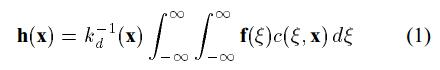1/kd(x)为归一化参数，保证绝对平滑的位置灰度值不变。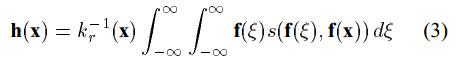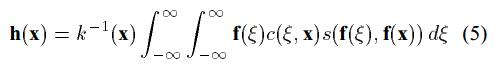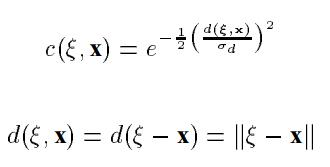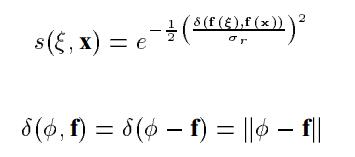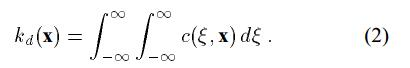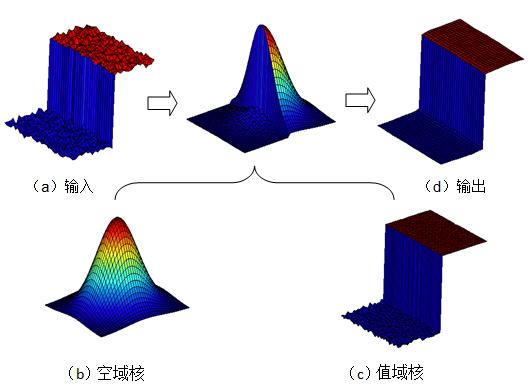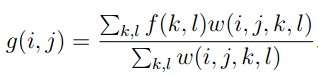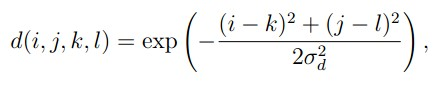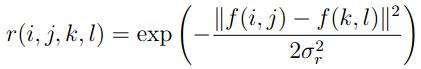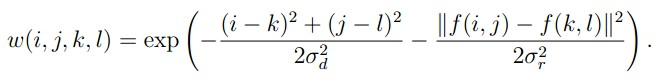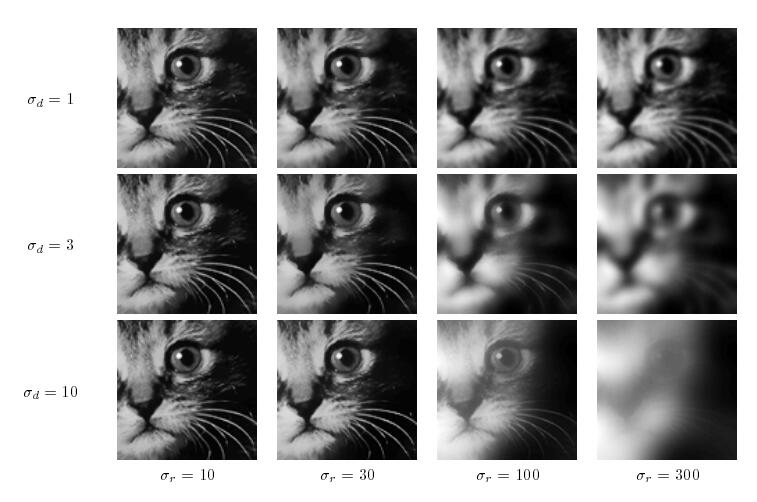void CShowPicView::OnBilateralFilter() // 双边滤波
{
CShowPicDoc* pDoc = GetDocument();
CDC* pDC=GetDC();
// 字符串
CString str;
int x,y;
int i,j,k,l;
double W_ijkl, D_ijkl, R_ijkl;
double Sum_W_ijkl;
double delta_d=3,delta_r=100;//空间位置方差，亮度方差
unsigned char g={0};//存放输出图像的像素值
double Sum_Data;

// 滤波器的高度
int iFilterH= 11;
// 滤波器的宽度
int iFilterW= 11;
// 中心元素的X坐标
int iFilterMX= 5;
// 中心元素的Y坐标
int iFilterMY= 5;

int lHeight=intHeight;//图像的宽
int lWidth=intWidth;//图像的宽

// 行(除去边缘几行)
for(i = iFilterMY; i < lHeight - iFilterH + iFilterMY + 1; i++)
{
// 列(除去边缘几列)
for(j = iFilterMX; j < lWidth - iFilterW + iFilterMX + 1; j++)
{
Sum_W_ijkl=0;//图像中心位置移动，置零
Sum_Data=0;

for (k = i-5; k < iFilterH+i-5; k++)
{
for (l = j-5; l < iFilterW+j-5; l++)
{
D_ijkl = exp(-1 * (pow(k-i,2)+pow(l-j,2))/(2*delta_d*delta_d) );
R_ijkl = exp(-1 *  pow(Data[k][l]-Data[i][j],2)/(2*delta_r*delta_r) );
W_ijkl = D_ijkl*R_ijkl;

Sum_Data+=W_ijkl*Data[k][l];
Sum_W_ijkl += W_ijkl;//权重累加和
}
}
g[i][j]=Sum_Data/Sum_W_ijkl;
}
}

for(y = 0; y < intHeight-1; y++)
for(int x = 0; x < intWidth-1; x++)
pDC->SetPixel(x+1*intWidth+30,y,RGB(g[y][x],g[y][x],g[y][x])); //打点显示图像
}

2017-06-21 16:48:57 qq_33854260 阅读数 458=======================================================================

% Douglas R. Lanman, Brown University, September 2006.
% dlanman@brown.edu, http://mesh.brown.edu/dlanman

[cpp] view plain
1. %简单地说:
2. %A为给定图像，归一化到[0,1]的矩阵
3. %W为双边滤波器（核）的边长/2
4. %定义域方差σd记为SIGMA(1),值域方差σr记为SIGMA(2)
5.
6. %%%%%%%%%%%%%%%%%%%%%%%%%%%%%%%%%%%%%%%%%%%%%%%%%%%%%%%%%%%%%
7. % Pre-process input and select appropriate filter.
8. function B = bfilter2(A,w,sigma)
9.
10. % Verify that the input image exists and is valid.
11. if ~exist('A','var') || isempty(A)
12.    error('Input image A is undefined or invalid.');
13. end
14. if ~isfloat(A) || ~sum([1,3] == size(A,3)) || ...
15.       min(A(:)) < 0 || max(A(:)) > 1
16.    error(['Input image A must be a double precision ',...
17.           'matrix of size NxMx1 or NxMx3 on the closed ',...
18.           'interval [0,1].']);
19. end
20.
21. % Verify bilateral filter window size.
22. if ~exist('w','var') || isempty(w) || ...
23.       numel(w) ~= 1 || w < 1
24.    w = 5;
25. end
26. w = ceil(w);
27.
28. % Verify bilateral filter standard deviations.
29. if ~exist('sigma','var') || isempty(sigma) || ...
30.       numel(sigma) ~= 2 || sigma(1) <= 0 || sigma(2) <= 0
31.    sigma = [3 0.1];
32. end
33.
34. % Apply either grayscale or color bilateral filtering.
35. if size(A,3) == 1
36.    B = bfltGray(A,w,sigma(1),sigma(2));
37. else
38.    B = bfltColor(A,w,sigma(1),sigma(2));
39. end
40.
41.
42. %%%%%%%%%%%%%%%%%%%%%%%%%%%%%%%%%%%%%%%%%%%%%%%%%%%%%%%%%%%%%
43. % Implements bilateral filtering for grayscale images.
44. function B = bfltGray(A,w,sigma_d,sigma_r)
45.
46. % Pre-compute Gaussian distance weights.
47. [X,Y] = meshgrid(-w:w,-w:w);
48. %创建核距离矩阵，e.g.
49. %  [x,y]=meshgrid(-1:1,-1:1)
50. %
51. % x =
52. %
53. %     -1     0     1
54. %     -1     0     1
55. %     -1     0     1
56. %
57. %
58. % y =
59. %
60. %     -1    -1    -1
61. %      0     0     0
62. %      1     1     1
63. %计算定义域核
64. G = exp(-(X.^2+Y.^2)/(2*sigma_d^2));
65.
66. % Create waitbar.
67. h = waitbar(0,'Applying bilateral filter...');
68. set(h,'Name','Bilateral Filter Progress');
69.
70. % Apply bilateral filter.
71. %计算值域核H 并与定义域核G 乘积得到双边权重函数F
72. dim = size(A);
73. B = zeros(dim);
74. for i = 1:dim(1)
75.    for j = 1:dim(2)
76.
77.          % Extract local region.
78.          iMin = max(i-w,1);
79.          iMax = min(i+w,dim(1));
80.          jMin = max(j-w,1);
81.          jMax = min(j+w,dim(2));
82.          %定义当前核所作用的区域为(iMin:iMax,jMin:jMax)
83.          I = A(iMin:iMax,jMin:jMax);%提取该区域的源图像值赋给I
84.
85.          % Compute Gaussian intensity weights.
86.          H = exp(-(I-A(i,j)).^2/(2*sigma_r^2));
87.
88.          % Calculate bilateral filter response.
89.          F = H.*G((iMin:iMax)-i+w+1,(jMin:jMax)-j+w+1);
90.          B(i,j) = sum(F(:).*I(:))/sum(F(:));
91.
92.    end
93.    waitbar(i/dim(1));
94. end
95.
96. % Close waitbar.
97. close(h);
98.
99.
100. %%%%%%%%%%%%%%%%%%%%%%%%%%%%%%%%%%%%%%%%%%%%%%%%%%%%%%%%%%%%%
101. % Implements bilateral filter for color images.
102. function B = bfltColor(A,w,sigma_d,sigma_r)
103.
104. % Convert input sRGB image to CIELab color space.
105. if exist('applycform','file')
106.    A = applycform(A,makecform('srgb2lab'));
107. else
108.    A = colorspace('Lab<-RGB',A);
109. end
110.
111. % Pre-compute Gaussian domain weights.
112. [X,Y] = meshgrid(-w:w,-w:w);
113. G = exp(-(X.^2+Y.^2)/(2*sigma_d^2));
114.
115. % Rescale range variance (using maximum luminance).
116. sigma_r = 100*sigma_r;
117.
118. % Create waitbar.
119. h = waitbar(0,'Applying bilateral filter...');
120. set(h,'Name','Bilateral Filter Progress');
121.
122. % Apply bilateral filter.
123. dim = size(A);
124. B = zeros(dim);
125. for i = 1:dim(1)
126.    for j = 1:dim(2)
127.
128.          % Extract local region.
129.          iMin = max(i-w,1);
130.          iMax = min(i+w,dim(1));
131.          jMin = max(j-w,1);
132.          jMax = min(j+w,dim(2));
133.          I = A(iMin:iMax,jMin:jMax,:);
134.
135.          % Compute Gaussian range weights.
136.          dL = I(:,:,1)-A(i,j,1);
137.          da = I(:,:,2)-A(i,j,2);
138.          db = I(:,:,3)-A(i,j,3);
139.          H = exp(-(dL.^2+da.^2+db.^2)/(2*sigma_r^2));
140.
141.          % Calculate bilateral filter response.
142.          F = H.*G((iMin:iMax)-i+w+1,(jMin:jMax)-j+w+1);
143.          norm_F = sum(F(:));
144.          B(i,j,1) = sum(sum(F.*I(:,:,1)))/norm_F;
145.          B(i,j,2) = sum(sum(F.*I(:,:,2)))/norm_F;
146.          B(i,j,3) = sum(sum(F.*I(:,:,3)))/norm_F;
147.
148.    end
149.    waitbar(i/dim(1));
150. end
151.
152. % Convert filtered image back to sRGB color space.
153. if exist('applycform','file')
154.    B = applycform(B,makecform('lab2srgb'));
155. else
156.    B = colorspace('RGB<-Lab',B);
157. end
158.
159. % Close waitbar.
160. close(h);

[cpp] view plain
2. I=double(I)/255;
3.
4. w     = 5;       % bilateral filter half-width
5. sigma = [3 0.1]; % bilateral filter standard deviations
6.
7. I1=bfilter2(I,w,sigma);
8.
9. subplot(1,2,1);
10. imshow(I);
11. subplot(1,2,2);
12. imshow(I1)2019-08-17 22:40:39 csuwoshikunge 阅读数 519

## 图像滤波问题描述

为了内容的完整性，首先对图像滤波进行简单的回顾。令$I\in R^{h\times w\times c}$表示图像，是一个二维矩阵，$h,w$分别表示图像的高度和宽度，$c=1,3$分别表示灰度图像和彩色图像，$I$中的每个元素$I(i,j)$称作一个像素。不失一般性，我们以$c=1$即灰度图为例。本质上图像$I$是一个定义二维网格点上的函数，也即$I:(i,j)\in R^{2} \rightarrow I(i,j)\in R$
$f:I\in R^{h\times w}\rightarrow \hat{I}\in R^{h\times w}$表示滤波器。常见的滤波过程就是：计算每个像素$I(i,j)$经过滤波后的值$\hat{I}(i,j)$，都是通过加权像素$I(i,j)$的邻域像素值，如下公式所示：
$\hat{I}(i,j)=f\circ I(i,j)=\frac{1}{\sum w(i',j')}\sum_{(i',j')\in N} w(i',j')I(i',j')\tag{1}$
其中$N$表示像素$I(i,j)$的邻域（也称滤波器的窗口），注意这里的邻域关系仅仅是像素点在定义域的相邻关系，如图像上的8邻域等。

## 1. 空间(即图像定义域)相邻关系的滤波器

常见的滤波器，如高斯滤波器，均值滤波器等，都属于这一类滤波器。
如(1)式所示，当权重
$w(i',j')=\frac{1}{n}$其中$n=|N|$表示邻域像素的个数， 这就均值滤波器。
当权重
$w(i',j')=\frac{1}{2\pi\sigma^2}e^{-{\frac{(i'-i)^2+(j'-j)^2}{2\sigma^2}}}$
就是通常的高斯滤波器。

注意这类滤波器，在进行图像滤波的时候仅仅考虑图像的定义域上的邻域关系(也即空间上的邻域关系)。这样通常会导致图像模糊，因为仅仅按图像定义域或空间上的相邻关系，会导致空间(或定义域)上相邻但是像素值相差巨大的像素参与滤波过程，如下图所示：按8邻域关系，计算点$A$的高斯滤波或均值滤波时，空间相邻的像素点$B,C,D,E$（注意它们的像素值（白）与$A$点的像素值（黑）相差很大），会参与滤波公式(1)中的加权平均。很显然，滤波后会导致，在$A$点附近的图像边缘会出现模糊。下面就介绍双边滤波，能够在很大程度上避免这一种仅仅依靠图像定义域相邻引起的图像模糊。

## 2. 双边滤波器（bilateral filter）

如何将上图中的点$A$$B,C,D,E$区分开来，使得(1)式中计算$A$点的加权平均时，$B,C,D,E$贡献的权重为0（当然这是理想的情况），就成了关键。那么一个很自然的想法是，同时考虑像素点的像素值，这样将像素点投到高维空间，那么有可能$B,C,D,E$将不再是$A$的相邻点。因为在低维空间中不易区分的点，在高维空间中可能比较容易区分开来。这点类似SVM（支持向量机）中的核函数设置的思想。

同时统计像素点$A$的坐标和像素值，这其实就是图像函数$I:(i,j)\rightarrow I(i,j)\in R$的graph, 记作
$G=\{(i,j,I(i,j)):(i,j)是图像像素坐标\}\tag{2}$
它是一个二维流形（嵌入在三位欧式空间$R^3$中），其实就是一个曲面，显然在这个graph $G$上，$B,C,D,E$$A$不再是相邻点。但是在直接二维流形$G$上寻找点的相邻点可能非常复杂，因为曲面的形状可能很不规则（曲率不为0），不像二维平面网格点$(i,j)$那样容易寻找相邻点。

为了计算方便，退而求其次，把二维曲面$G$嵌入在三维空间中考虑，利用三维欧式空间$R^3$的曲率为0，在三维网格点中寻找相邻点，这样就和在二维网格中种寻找相邻点一样方便。但是考虑到嵌入的$G$实则是函数$I$的图像graph，本质上是二维的，所以双边滤波仍按定义域寻找相邻点即可，但是要减弱这些相邻点中颜色差异较大的点对滤波贡献的权重。

可以看到，在把$G$嵌入三维空间考虑时，$A$点在对应$(i_A,j_A,I_A)$。以$D$点为例，$D$对应$(i_D,j_D,I_D)$。不妨以欧式范数为例，在三维空间中$A,D$之间的距离为
$d(A,D)=\sqrt{(i_D-i_A)^2+(j_D-j_A)^2+(I_D-I_A)^2}=\sqrt{1+1+(I_D-I_A)^2}\tag{3}$
则如上面(3)式所示，那么当$A,D$的像素值之差$|I_D-I_A|$很大时，那么在$G$$d(A,D)$距离就很远。
而双边滤波正是通过，考虑这种距离来弱化$D$$A$的影响，这点可以从下面双边滤波的公式中，得到体现
$\hat{I}_A=\frac{1}{\sum w(q,A)}\sum_{q\in N}w(q,A)I_q\tag{4}$
其中，$N$是点$A$的邻域，
$w(q,A)=e^{-\frac{(i_q-i_A)^2+(j_q-j_A)^2}{2\sigma_{s}^{2}}-\frac{(I_q-I_A)^2}{2\sigma_{r}^2}}$

显然，当$q,A$之间的像素值相差很大时，$w(q,A)$就会变得很小，从而达到了弱化$q$点对$A$点的滤波值的影响，易见上图中的$B,C,D,E$$A$的距离很大（在$R^3$中考虑它们之间的距离时）。所以滤波时，$B,C,D,E$$A$的影响就大大减弱，从而大大减轻滤波后边界模糊的现象，达到了保边滤波的效果。

## 3. 如何快速实现双边滤波

即使双边滤波有很好的保边效果，但是当图像的尺寸以及滤波器的窗口尺寸很大时，双边滤波的速度就可能很慢。双边滤波这不像通常的高斯滤波和均值率波等，双边滤波(4)式中的权重系数$w$是与图像的像素值有关的，因而不能像高斯滤波或者均值滤波那样有固定的模板通过卷积快速实现。后面系列，会介绍快速实现近似双边滤波的相关文章以及在双边滤波在深度学习中的应用。。。。。

: Fast high-dimensional filtering using the permutohedral lattice.

图像处理双边滤波器 相关内容

2015-03-16 15:41:36 u014568921 阅读数 4863

双边滤波（Bilateral filter）是一种非线性的滤波方法，是结合图像的空间邻近度和像素值相似度的一种折衷处理，同时考虑空域信息和灰度相似性，达到保边去噪的目的。普通的高斯滤波会将图像的边缘模糊掉，而双边滤波器具有保边特性。

一般的高斯模糊在进行采样时主要考虑了像素间的空间距离关系，但是却并没有考虑像素值之间的相似程度，因此这样我们得到的模糊结果通常是整张图片一团模糊。Bilateral blur的改进就在于在采样时不仅考虑像素在空间距离上的关系，同时加入了像素间的相似程度考虑，因而可以保持原始图像的大体分块进而保持边缘。

下面先讲高斯滤波，明白的同学请直接跳过。

## 高斯滤波

在2D高斯滤波中的具体实现就是对周围的一定范围内的像素值分别赋以不同的高斯权重值，并在加权平均后得到当前点的最终结果。而这里的高斯权重因子是利用两个像素之间的空间距离（在图像中为2D）关系来生成。通过高斯分布的曲线可以发现，离目标像素越近的点对最终结果的贡献越大，反之则越小。

高斯核函数一种最常用的径向基函数,形式为其中xc为核函数中心,σ为函数的宽度参数 , 控制了函数的径向作用范围。所谓径向基函数 (Radial Basis Function 简称 RBF), 就是某种沿径向对称的标量函数 通常定义为空间中任一点x到某一中心xc之间欧氏距离的单调函数 , 可记作 k(||x-xc||), 其作用往往是局部的 , 即当x远离xc时函数取值很小。
高斯函数具有五个重要的性质，这些性质使得它在早期图像处理中特别有用．这些性质表明，高斯平滑滤波器无论在空间域还是在频率域都是十分有效的低通滤波器，且在实际图像处理中得到了工程人员的有效使用．高斯函数具有五个十分重要的性质，它们是：
（1）二维高斯函数具有旋转对称性，即滤波器在各个方向上的平滑程度是相同的．一般来说，一幅图像的边缘方向是事先不知道的，因此，在滤波前是无法确定一个方向上比另一方向上需要更多的平滑．旋转对称性意味着高斯平滑滤波器在后续边缘检测中不会偏向任一方向．
（2）高斯函数是单值函数．这表明，高斯滤波器用像素邻域的加权均值来

代替该点的像素值，而每一邻域像素点权值是随该点与中心点的距离单调增减的．这一性质是很重要的，因为边缘是一种图像局部特征，如果平滑运算对离算子中心很远的像素点仍然有很大作用，则平滑运算会使图像失真．

（3）高斯函数的付立叶变换频谱是单瓣的．正如下面所示，这一性质是高斯函数付立叶变换等于高斯函数本身这一事实的直接推论．图像常被不希望的高频信号所污染(噪声和细纹理)．而所希望的图像特征（如边缘），既含有低频分量，又含有高频分量．高斯函数付立叶变换的单瓣意味着平滑图像不会被不需要的高频信号所污染，同时保留了大部分所需信号．

（4）高斯滤波器宽度(决定着平滑程度)是由参数σ表征的，而且σ和平滑程度的关系是非常简单的．σ越大，高斯滤波器的频带就越宽，平滑程度就越好．通过调节平滑程度参数σ，可在图像特征过分模糊(过平滑)与平滑图像中由于噪声和细纹理所引起的过多的不希望突变量(欠平滑)之间取得折衷．

（5）由于高斯函数的可分离性，大高斯滤波器可以得以有效地实现．二维高斯函数卷积可以分两步来进行，首先将图像与一维高斯函数进行卷积，然后将卷积结果与方向垂直的相同一维高斯函数卷积．因此，二维高斯滤波的计算量随滤波模板宽度成线性增长而不是成平方增长．

## 高斯滤波的问题

高斯滤波没有考虑图像的边缘，会将边缘模糊掉。因为高斯核只考虑了空间分布，没有考虑到像素值的差异。图像的边缘往往是图像灰度剧烈变化的地方。所以可以综合考虑图像灰度因素。

## 双边滤波器原理

双边滤波方法(Bilateral filtering)是基于Gauss 滤波方法提出的，主要是针对Gauss 滤波中将Gauss 权系数直接与图像信息作卷积运算进行图像滤波的原理，将滤波权系数优化成Gauss 函数和图像亮度信息的乘积，优化后的权系数再与图像信息作卷积运算，这样就能在滤波的同时考虑到图像信息中的图像边缘信息，使图像在正常Gauss 滤波后很模糊的边缘信息得以保持清晰，并且图像边缘更加平滑。此方法对于彩色和灰度图像的滤波均适用，具有很强的实用性。
设原图为f(x,y),(x,y)为像素的坐标，双边滤波后(x,y)点的像素值变为公式中Sx,y 表示中心点（x,y）的（2N+1）*（2N+1）大小的领域。实际上，公式右边就是中心像素点邻域内像素亮度值的加权平均。
权值W由两部分组成W(i,j)=Ws(i,j)*Wr(i,j)
双边滤波器的加权系数是这两部分因子的非线性组合，空间邻近度因子Ws和亮度相似度因子Wr的乘积。前者随着像素点与中心点之间欧几里德距离的增加而减小，后者随着两像素亮度值之差的增大而减小。在图像变化平缓的区域，邻域内像素亮度值相差不大，双边滤波转化为高斯低通滤波器；在图像变化剧烈的区域，滤波器利用边缘点附近亮度值相近的像素点的亮度值平均代替原亮度值。因此，双边滤波器既平滑滤波了图像，又保持了图像的边缘。双边滤波器受3个参数的控制：滤波器半宽N、参数δs和δr。N越大，平滑作用越强；δs和δr分别控制着空间邻近度因子Ws和亮度像似度因子Wr的衰减程度。

#define  MAX_IMAGE_SIZE 1024
double d[MAX_IMAGE_SIZE][MAX_IMAGE_SIZE];//d[i][j]表示入图像，fi][j]表示出图像。
double f[MAX_IMAGE_SIZE][MAX_IMAGE_SIZE];
void CImageColorProcess::Bilateral(LPBYTE lpSrc, LPBYTE lpDst, int nSrcCount, int nW, int nH)
{
int i, j, k, l;
int p = 5;//p决定模板大小。当p=1时，模板为3*3;当p=2时，模板为5*5;当p=n时，模板为(2n+1)*(2n+1)。
short m, n;
double a1 = 0.02, b1 = 0.002;
double aa1, bb1;//aa1,bb1为滤波模板中的各权重。
//gguiyi高斯滤波的归一化系数，bguiyi双边滤波的归一化系数，gsum高斯滤波像素的加权和，bsum双边滤波像素的加权和。
double gguiyi = 0.0, bguiyi = 0.0, gsum = 0.0, bsum = 0.0;
int x_size2 = nW;//x_size1;
int y_size2 = nH;//y_size1;
LPBYTE lpSrc1 = new byte[nW*nH];
RGB2Gray(lpSrc, lpSrc1, 24, nW, nH);
//将读取的噪声图像赋给入力图像
for (i = 0; i < nH; i++)
{
for (j = 0; j < nW; j++)
{
d[i][j] = lpSrc1[i*nW + j];
}
}
//高斯滤波，双边滤波处理噪声图像
for (i = 0; i < nH; i++)
{
for (j = 0; j < nW; j++)
{
for (k = -p; k <= p; k++)
{
for (l = -p; l <= p; l++)
{
m = i + k; n = j + l;
//abs()返回指定数字的绝对值
if (m<0)  { m = abs(i + k) - 1; }   	   
<span style="font-size: 12px;">                                        </span><span style="font-family: Arial, Helvetica, sans-serif; font-size: 12px;"> if (m>nH - 1) { m = 2 * nH - i - k - 1; }</span>
					if (n<0)  { n = abs(j + l) - 1; }	  if (n>nW - 1) { n = 2 * nW - j - l - 1; }
aa1 = exp(-a1*(l*l + k*k));
bb1 = exp(-b1*(d[i][j] - d[m][n])*(d[i][j] - d[m][n]));
bsum += aa1*bb1*d[m][n];
bguiyi += aa1*bb1;
}
}
f[i][j] = bsum / bguiyi;
gguiyi = 0.0, bguiyi = 0.0, gsum = 0.0, bsum = 0.0;
}
}
//将滤波后的像素赋给新的图像
for (i = 0; i < nH; i++)
{
for (j = 0; j < nW; j++)
{
lpDst[i*nW + j] = (unsigned char)(f[i][j] + 0.5);
}
}

}

效果以上三图从上到下分别为原图，高斯模糊和双边滤波后的效果图，双边滤波的保边效果还是很明显的。

图像处理双边滤波器 相关内容

2019-08-29 22:34:39 charce_you 阅读数 110

## 6.1 图像过滤

### 6.1.1 cv::bilateralFilter

6.1.1.1 双边滤波器简介

在图像处理上，双边滤波器为使影像平滑化的非线性滤波器。和传统的影像平滑化算法不同，双边滤波器除了使用像素之间几何上的靠近程度之外，还多考虑了像素之间的光度/色彩差异， 使得双边滤波器能够有效的将影像上的噪声去除，同时保存影像上的边缘资讯。
双边滤波器可以应用在影像降噪、色调映射、图像重照明和材质编辑。
色调映射是在有限动态范围媒介上近似显示高动态范围图像的一项7图形学技术。打印结果、CRT 或者 LCD 显示器以及投影仪等都只有有限的动态范围。 本质上来讲，色调映射是要解决的问题是进行大幅度的对比度衰减将场景亮度变换到可以显示的范围，同时要保持图像细节与颜色等对于表现原始场景非常重要的信息。 因此，双边滤波器可以借由对亮度相似区域进行模糊化之后，将高动态范围图像的梯度图像分割成亮度相似区和亮度差异较大的部分，借由改变两者的权重，而将高动态范围图像映射成可显示的有限动态范围。

6.1.1.2 Opencv接口

void cv::bilateralFilter(InputArray src,
OutputArray dst,
int 	d,
double 	sigmaColor,
double 	sigmaSpace,
int 	borderType = BORDER_DEFAULT
)
参数
src	源8位或浮点，1通道或3通道图像。
dst	与src具有相同大小和类型的目标图像。
d	过滤期间使用的每个像素邻域的直径。如果它是非正数，则从sigmaSpace计算。
sigmaColor	过滤颜色空间中的西格玛。参数的值越大意味着像素邻域内的更远的颜色（参见sigmaSpace）将混合在一起，从而产生更大的半等颜色区域。
sigmaSpace	在坐标空间中过滤西格玛。较大的参数值意味着只要它们的颜色足够接近，更远的像素就会相互影响（参见sigmaColor）。当d> 0时，无论sigmaSpace如何，它都指定邻域大小。否则，d与sigmaSpace成比例。
borderType 	用于外推图像外部像素的边框模式，请参阅BorderTypes

该功能将双边滤波应用于输入图像，减少不需要的噪声，同时保持边缘相当清晰。但是，与大多数过滤器相比，它非常慢。
Sigma值：为简单起见，您可以将2 sigma值设置为相同。如果它们很小（<10），过滤器将没有太大的影响，而如果它们很大（> 150），它们将具有非常强烈的效果，使图像看起来“卡通”。
滤波器大小：大滤波器（d> 5）非常慢，因此建议对实时应用使用d = 5，对于需要大量噪声滤波的离线应用，可能需要d = 9。

void ES::ImageProcessing::bilateralOper(cv::Mat* dst)
{
cv::resize(src, src, Size(src.rows / 4 * 3, src.cols / 4 * 3));
ImageProcessingParams* img_params = stat-ic_cast<ImageProcessingParams*>(m_params);
int d = img_params->m_d;//4
int sigmaColor = img_params->m_sigmaColor;//183
int sigmaSpace = img_params->m_sigmaSpace;//188
Mat mat;
cv::bilateralFilter(src, mat, d, sigmaColor, sigmaSpace);
Mat mergeMat(src.rows, src.cols + mat.cols, src.type());
Mat submat = mergeMat.colRange(0, src.cols);
src.copyTo(submat);
submat = mergeMat.colRange(src.cols, src.cols + mat.cols);
mat.copyTo(submat);
mergeMat.copyTo(*dst);
}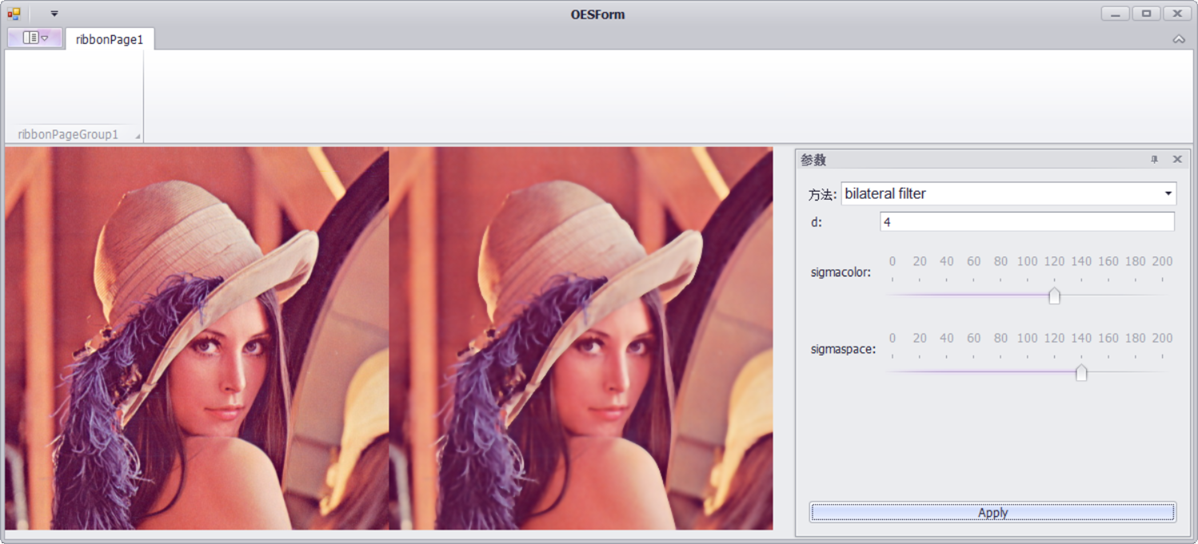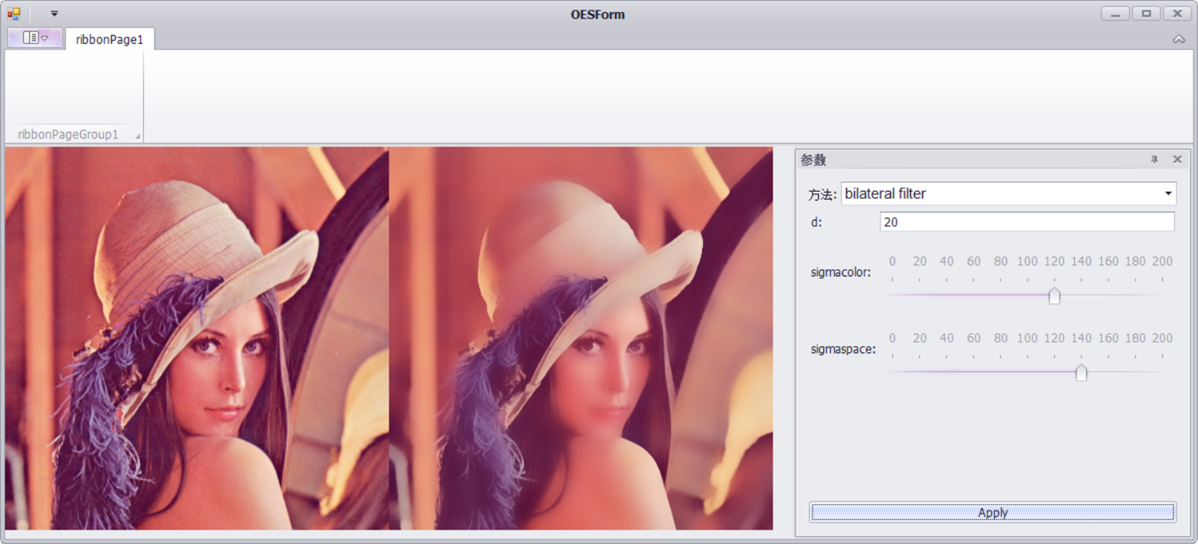图像处理双边滤波器 相关内容

阅读数 1192

博文 来自： xinanzhung

阅读数 5983

阅读数 1633

博文 来自： GarfieldEr007

阅读数 6680

博文 来自： lxy201700

阅读数 6650

博文 来自： cyh706510441
没有更多推荐了，返回首页